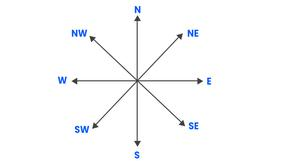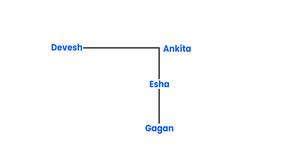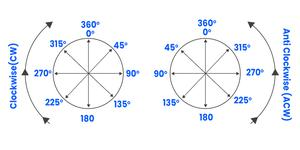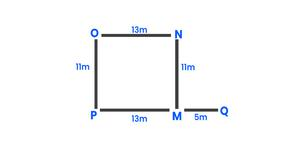# Direction and Distance: Concepts, Questions, Solved Examples

• Last Updated : 03 Feb, 2022

There are many different types of questions that are asked from the Direction and Distance topic. Those questions are generally related to the direction and distance between two or more persons, products or elements, etc. Thus, many different types of questions can be formed based on the position of any person, thing, or product. In this topic, we will discuss how the concepts of Direction and Distance works. Let us understand, it with the help of the important rules:

There are four cardinal directions North (N), South (S), East (E), and West (W), and the four ordinal directions (also called the intercardinal direction) North-East (NE), North-West (NW), South-East (SE), and South -West (SW).### Tips and Tricks:

• In case a person facing east, on taking left he will face towards the north and on taking the right turn he will face towards the south.
• In case a person facing west, on taking left he will face towards the south and on taking right turn he will face towards the north.
• In case a person facing north, on taking left he will face towards the west and on taking the right turn he will face towards the east.
• In case a person facing south, on taking left he will face towards the east and on taking the right turn he will face towards the west.

### a) Basic Direction based question:

Q1. Ankita is in the east of Devesh and in the north of Esha. If Gagan is in the south of Esha, then Ankita is in which direction with respect to Gagan?

Solution:

let us understand it with the help of diagrams.Let us start with Esha, and then from there we can draw others position as well.

Hence, Ankita is towards the North of Gagan.

### b) Degree based questions:

• If a person goes to his left side, he will go towards the anti-clockwise direction.
• If a person goes to his right side, he will go towards the clockwise direction.

* In case of moving towards left or right side, we assume that the movement is at an angle of 90 degrees.in easy words  we can say that:

Left turn = Anticlockwise turn

Right turn = Clockwise turn.

Q2. Anamika is facing north. She turns 135 degree in the anticlockwise direction and then another 180 degree in the same direction. Find which direction she is facing now?

1) East

2) West

3) North

4) South-East

5) North-East

Solution:

According to the question, she turns 135 degree in the anticlockwise direction and then another 180 degree in the same direction

Therefore, she turns total (135 + 180) = 315 degree anticlockwise

Hence, she turns 315 degree to her left side.

In the primary stage if she faces north then, finally she will face the North-East direction.

### c) Coded Direction based question:

In such types of questions, the directions are given in a certain coded language.

3Q. Direction: Study the following information carefully and answer the questions given below.

In a coded language:

• A@B (11) means A is 11m in the north of B
• A*B (26) means A is 26m in the south of B
• A&B (18) means A is 18m in the east of B
• A~B (21) means A is 21m in the west of B

N@M (11), O~N (13), P*O (11), Q&P (18); Then, M is in which direction with respect to Q?

1) North

2) West

3) North-west

4) East

5) South-east

Solution:M is in the west direction with respect to Q.

Note: To find the Shortest Distance between any two points:

Use the formula of Pythagoras theorem i.e., H^2 = √(P^2+B^2 )

My Personal Notes arrow_drop_up